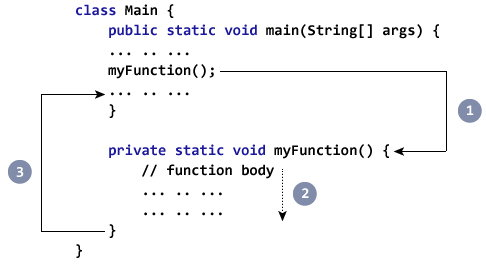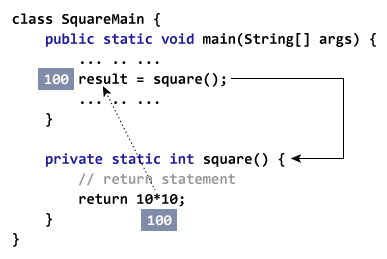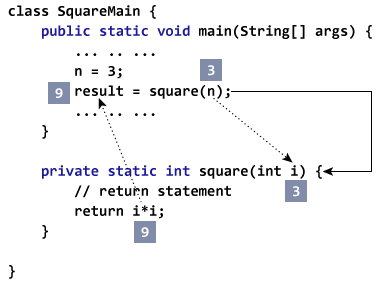Provide Best Programming Tutorials

## How to call a Java Method?

### How to call a Java Method?

Now you defined a method, you need to use it. For that, you have to call the method. Here’s how:

`myMethod();`

This statement calls the `myMethod()` method that was declared earlier.1. While Java is executing the program code, it encounters `myMethod();` in the code.

2. The execution then branches to the `myFunction()` method, and executes code inside the body of the method.

3. After the codes execution inside the method body is completed, the program returns to the original state and executes the next statement.

### Example: Complete Program of Java Method

Let’s see a Java method in action by defining a Java class.

```class Main {
​
public static void main(String[] args) {
​
// method call
myMethod();
​
System.out.println("Method was executed successfully!");
}
​
// method definition
private static void myMethod(){
System.out.println("Printing from inside myMethod()!");
}
}```

When you run the program, the output will be:

```About to encounter a method.
Printing from inside myMethod().
Method was executed successfully!```

The method `myMethod()` in the above program doesn’t accept any arguments. Also, the method doesn’t return any value (return type is `void`).

Note that, we called the method without creating object of the class. It was possible because `myMethod()` is static.

Here’s another example. In this example, our method is non-static and is inside another class.

```class Main {
​
public static void main(String[] args) {
​
Output obj = new Output();
​
// calling myMethod() of Output class
obj.myMethod();
​
System.out.println("Method was executed successfully!");
}
}
​
class Output {

// public: this method can be called from outside the class
public void myMethod() {
System.out.println("Printing from inside myMethod().");
}
}```

When you run the program, the output will be:

```About to encounter a method.
Printing from inside myMethod().
Method was executed successfully!```

Note that, we first created instance of Output class, then the method was called using objobject. This is because `myMethod()` is a non-static method.

### Java Methods with Arguments and Return Value

A Java method can have zero or more parameters. And, they may return a value.

### Example: Return Value from Method

Let’s take an example of method returning a value.

```class SquareMain {
public static void main(String[] args) {
int result;
result = square();
System.out.println("Squared value of 10 is: " + result);
}
​
public static int square() {
// return statement
return 10 * 10;
}
​
}```

When you run the program, the output will be:

`Squared value of 10 is: 100`

In the above code snippet, the method `square()` does not accept any arguments and always returns the value of 10 squared.

Notice, the return type of `square()` method is `int`. Meaning, the method returns an integer value.As you can see, the scope of this method is limited as it always returns the same value.

Now, let’s modify the above code snippet so that instead of always returning the squared value of 10, it returns the squared value of any integer passed to the method.

### Example: Method Accepting Arguments and Returning Value

```public class SquareMain {

public static void main(String[] args) {
int result, n;

n = 3
result = square(n);
System.out.println("Square of 3 is: " + result);

n = 4
result = square(n);
System.out.println("Square of 4 is: " + result);
}
​
static int square(int i) {
return i * i;
}
}```

When you run the program, the output will be:

```Squared value of 3 is: 9
Squared value of 4 is: 16```

Now, the `square()` method returns the squared value of whatever integer value passed to it.Java is a strongly-typed language. If you pass any other data type except `int` (in the above example), compiler will throw an error.

The argument passed n to the `getSquare()` method during the method call is called actual argument.

`result = getSquare(n);`

The parameter i accepts the passed arguments in the method definition `getSquare(int i)`. This is called formal argument (parameter). The type of the formal argument must be explicitly typed.

You can pass more than one argument to the Java method by using commas. For example,

```public class ArithematicMain {
​
public static int getIntegerSum (int i, int j) {
return i + j;
}
​
public static int multiplyInteger (int x, int y) {
return x * y;
}
​
public static void main(String[] args) {
System.out.println("10 + 20 = " + getIntegerSum(10, 20));
System.out.println("20 x 40 = " + multiplyInteger(20, 40));
}
}```

When you run the program, the output will be:

```10 + 20 = 30
20 x 40 = 800```

The data type of actual and formal arguments should match, i.e., the data type of first actual argument should match the type of first formal argument. Similarly, the type of second actual argument must match the type of second formal argument and so on.